XLL+ Class Library (7.0)

# Importing a .NET Assembly

This walkthrough demonstrates how to import a .NET assembly, and automatically generate Excel add-in functions for its methods and properties.

You can find a copy of all the code used in this walkthrough in the `Walkthroughs/NetWrap` folder in your XLL+ installation, along with a demonstration spreadsheet, `NetWrap.xls`.

### Run-time requirements

Please note that if you are using Visual Studio 2005, you must have Service Pack 1 installed in order to build a valid XLL.

If the add-in fails to load into Excel at run-time, please see the technical note .NET requirements.

In order to build `MyLib.dll`, you need the C# project system to be installed on your computer.

### Creating the add-in project

1. From the File menu, select New and then Project to open the New Project dialog.

2. Select the XLL+ 7 Excel Add-in project template from the list of Visual C++ Projects, and enter NetWrap in the Name box. Under Location, enter an appropriate directory in which to create the project.

3. Put a tick next to "Create directory for solution", and click OK.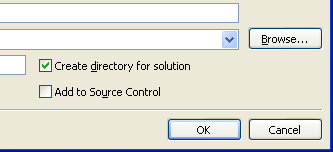4. In the "Application Settings" page of the XLL+ AppWizard, put a tick against Common Language Runtime Support (/clr).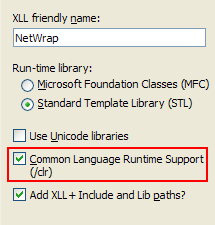5. Click Finish to create the new add-in project.

For more information about creating projects, see Creating an add-in project in the XLL+ User Guide.

### Creating the imported .NET assembly

Now create a new C# project and add it to the solution.

1. In the Visual Studio Solution Explorer window, right-click and select Add/New Project....

2. Select the Visual C# project type in the tree on the left-hand side, and select Class Library in the list on the right. (In Visual Studio 2017, select Class Library (.NET Framework).)

3. Enter `MyLib` for the project Name, and accept the default Location. Press OK.

4. Open the project properties of MyLib, and, in the Build page, towards the bottom of the page, put a tick against XML documentation file.

5. If you are using Visual Studio 2015, then select the Application page of the project properties, and set the Target framework to be `.NET Framework 4`. This will make the .NET project compatible with the XLL+ C++ project. Alternatively, you can change the C++ project to use .NET Framework 4.5 or above as its target framework. Close the project in Visual Studio, or unload it, and directly edit the project file `NetWrap.vcxproj`. Locate the element `<PropertyGroup Label="Globals">` and insert the desired target framework, e.g.: <TargetFrameworkVersion>v4.5.2</TargetFrameworkVersion> See How to: Modify the Target Framework and Platform Toolset for details.

6. Open `Class1.cs` and replace all the code with the code below.CopyC#
```using System;
using System.ComponentModel;

namespace MyLib
{
/// <summary>
/// Contains static calls to test parameter &amp; return types
/// </summary>
public static class TestClass
{
/// <summary>
/// returns the concatenation of the arguments.
/// </summary>
/// <param name="a">is a string</param>
/// <param name="b">is a number</param>
/// <param name="c">is an integer</param>
/// <param name="d">is a boolean</param>
/// <returns></returns>
public static string T1(string a, double b, int c, bool d)
{
return String.Format("a={0};b={1};c={2};d={3}",
a, b, c, d);
}
/// <summary>
/// returns an array of strings which are concatenations
/// of the arguments.
/// </summary>
/// <param name="a">is a vector of strings</param>
/// <param name="b">is a vector of numbers. It must be the same length as a.</param>
/// <param name="c">is a vector of integers. It must be the same length as a.</param>
/// <param name="d">is a vector of booleans. It must be the same length as a.</param>
public static string[] T2(string[] a, double[] b,
int[] c, bool[] d)
{
if (a.Length != b.Length)
throw new ArgumentException(String.Format("Expected {0} items for b", a.Length));
if (a.Length != c.Length)
throw new ArgumentException(String.Format("Expected {0} items for c", a.Length));
if (a.Length != d.Length)
throw new ArgumentException(String.Format("Expected {0} items for d", a.Length));
string[] res = new string[a.Length];
for (int i = 0; i < res.Length; i++)
res[i] = T1(a[i], b[i], c[i], d[i]);
return res;
}
/// <summary>
/// is a test function with
/// irritating
/// formatting.
/// </summary>
/// <param name="a">is partly this,
/// and partly that</param>
/// <param name="b">is &gt; <paramref name="a"/></param>
/// <returns></returns>
public static int T3(int a, int b)
{
return a + b;
}
/// <summary>
/// A test function that adds two numbers, with formatted comments,
/// some of which
/// are
/// on
/// <i>separate</i>
/// lines.
/// </summary>
/// <param name="a">is the first number</param>
/// <param name="b">is the second number</param>
/// <returns>the sum of the numbers</returns>
public static double T4(double a, double b)
{
return a + b;
}

[Description("is described in a ComponentModel description.")]
public static double T5(
[Description("is a matrix of numbers")] double[,] a,
[Description("is another matrix of numbers")] double[,] b)
{
return 0.0;
}

/// <summary>
/// A publicly visible enumerated type
/// </summary>
public enum MyEnum
{
Zero,
One,
Two,
Three
}
/// <summary>
/// Returns the value of an enum
/// </summary>
/// <param name="e">A value of type MyEnum</param>
/// <returns>The numeric value of e</returns>
public static double T6(MyEnum e)
{
return (double)(int)e;
}
/// <summary>
/// Returns the length of an array of MyEnum's.
/// </summary>
/// <param name="enums">An array of values of type MyEnum</param>
/// <returns>The length of the array</returns>
public static int T7(MyEnum[] enums)
{
return enums.Length;
}
/// <summary>
/// Returns a value of variable type
/// </summary>
/// <param name="type">controls the type of value returned</param>
/// <returns>an object, which is transformed to the appropriate Excel type by the generated code.</returns>
public static object T8(int type)
{
switch (type)
{
case 0:
return 123.45;
case 1:
return "A string";
case 2:
return true;
case 3:
return (Decimal)99.99;
case 10:
return new double[] { 1.2, 3.4, 5.6 };
case 11:
return new string[] { "The", "cat", "sat" };
case 12:
return new bool[] { true, false, false, true };
case 13:
return new Decimal[] { (Decimal)10.5, (Decimal)99999.90 };
case 14:
return new object[] { (double)123.45, true, "A string in an array" };
case 20:
{
double[,] res = new double[2, 3];
res[0, 0] = 0.0;
res[0, 1] = 0.1;
res[0, 2] = 0.2;
res[1, 0] = 1.0;
res[1, 1] = 1.1;
res[1, 2] = 1.2;
return res;
}
case 21:
{
string[,] res = new string[3, 2];
res[0, 0] = "One";
res[0, 1] = "Two";
res[1, 0] = "Three";
res[1, 1] = "Four";
res[2, 0] = "Five";
res[2, 1] = "Six";
return res;
}
case 22:
{
object[,] res = new object[2, 3];
res[0, 0] = 123.45;
res[0, 1] = "Two";
res[0, 2] = true;
res[1, 0] = (Decimal)99.99;
res[1, 1] = "Three";
res[1, 2] = false;
return res;
}
}
throw new ArgumentException("unexpected value for type", "type");
}
}

/// <summary>
/// A factory class for creating Things.
/// </summary>
public static class Factory
{
/// <summary>
/// Create a Thing using the default key.
/// </summary>
/// <returns></returns>
public static Thing CreateObject()
{
return CreateObject(Thing.DefaultKey);
}
/// <summary>
/// Create a Thing using the specified key.
/// </summary>
/// <param name="key">is a string key.</param>
public static Thing CreateObject(string key)
{
return new Thing(key);
}
}

/// <summary>
/// A class with properties and instance methods.
/// </summary>
public class Thing : IDisposable
{
/// <summary>
/// The key used if none is supplied.
/// </summary>
public const string DefaultKey = "a_default_key";
private string key;
/// <summary>
/// The key to the object
/// </summary>
public string Key
{
get { return key; }
set { key = value; }
}

/// <summary>
/// Returns the length of the object's key
/// </summary>
/// <returns></returns>
public int KeyLen()
{
return key.Length;
}

internal Thing(string key)
{
count++;
System.Diagnostics.Trace.WriteLine(String.Format(
"Constructing Thing({0}); count = {1}", key, count));
this.key = key;
}

/// <summary>
/// An indexed property
/// </summary>
/// <param name="index">A zero-based index into the key</param>
/// <returns></returns>
public string this[int index]
{
get
{
return key.Substring(index, 1);
}
}

/// <summary>
/// Compares two Things by value
/// </summary>
/// <param name="thing1">First thing</param>
/// <param name="thing2">Second thing</param>
/// <returns></returns>
public static int Compare(Thing thing1, Thing thing2)
{
if (thing1 == null)
return (thing2 == null) ? 0 : -1;
if (thing2 == null)
return 1;
return String.Compare(thing1.Key, thing2.Key);
}

/// <summary>
/// Concatenates the keys of a vector of Things
/// </summary>
/// <param name="things">Vector of Things</param>
/// <returns></returns>
public static string Combine(Thing[] things)
{
System.Text.StringBuilder sb = new System.Text.StringBuilder();
foreach (Thing t in things)
sb.Append(t.Key);
return sb.ToString();
}

/// <summary>
/// Finds the highest-sorted key in a matrix of Things
/// </summary>
/// <param name="things">2-dimensional array of things</param>
/// <returns></returns>
public static string MaxKey(Thing[,] things)
{
string max = "";
for (int i = 0; i < things.GetLength(0); i++)
{
for (int j = 0; j < things.GetLength(1); j++)
{
if (max == ""
|| String.Compare(things[i, j].Key, max) > 0)
max = things[i, j].Key;
}
}
return max;
}
/// <summary>
/// Takes untyped arguments
/// </summary>
/// <param name="scalarArg">An untyped value</param>
/// <param name="vectorArg">A vector of untyped values</param>
/// <param name="matrixArg">A matrix of untyped values</param>
/// <returns></returns>
public static object VarArgTest(object scalarArg, object[] vectorArg, object[,] matrixArg)
{
object[] result = new object[vectorArg.Length + 2];
result = scalarArg;
Array.Copy(vectorArg, 0, result, 1, vectorArg.Length);
result[result.Length - 1] = String.Format("{0} x {1}", matrixArg.GetLength(0), matrixArg.GetLength(1));
for (int i = 0; i < result.Length; i++)
if (result[i] == null)
result[i] = "";
return result;
}

/// <summary>
/// A public enumeration
/// </summary>
public enum Periods
{
Monthly = 12,
Quarterly = 4,
SemiAnnual = 2,
Annual = 1
}
/// <summary>
/// Calculates the discount factor for a given period and interest rate
/// </summary>
/// <param name="rate">is the interest rate</param>
/// <param name="period">is a time period</param>
/// <returns></returns>
public static double DiscountFactor(double rate, Periods period)
{
return Math.Exp((-1.0/((double)(int)period)) * rate);
}

/// <summary>
/// Returns a forex rate for the specified currency
/// </summary>
/// <param name="ccy">is an ISO currency code</param>
/// <returns></returns>
public static double GetSpotRate(string ccy)
{
switch (ccy)
{
case "USD": return 1.0;
case "JPY": return 85.34;
case "AUD": return 0.8916;
case "EUR": return 1.2725;
case "GBP": return 1.5566;
}
throw new ArgumentException("Unknown currency");
}

/// <summary>
/// A public shared count of existing objects.
/// </summary>
public static int count = 0;
void IDisposable.Dispose()
{
System.Diagnostics.Trace.WriteLine(String.Format(
"Disposing Thing({0}); count = {1}", key, count));
}

/// <summary>
/// The finalizer, called when the Thing is actually destroyed.
/// </summary>
~Thing()
{
count--;
System.Diagnostics.Trace.WriteLine(String.Format(
"Finalized Thing({0}); count = {1}", key, count));
}

}

}```

### Add a reference to the assembly

Before we can import the .NET assembly, we must create a reference to it.

1. In Solution Explorer, find the node for the project NetWrap and right-click it. Select Properties.

2. In the Project Properties window, select the node "Common Properties/References", and click the "Add New Reference..." button.

3. In Visual Studio 2015 or 2017, right-click the the "References" node beneath the project node and click "Add Reference...".

4. You may have to wait a long time for the "Add Reference" window to appear.

5. In the "Add Reference" window, select the tab "Projects", select "MyLib" in the list, and press OK.

6. In the Project Properties window, click OK to save your changes.

7. You should now build the solution (Shift+Ctrl+B), and make sure that both projects built successfully. Make sure that the build is set to "Win32", "x86" or "x64"; avoid "Mixed Platforms". Also, if necessary, use the Build/Configuration Manager... command to open the solution configuration window, and ensure that both projects have a tick in the "Build" column.

Note that on Visual Studio 2015 and 2017, where there is no "Common Properties/References" node in the Project Properties, you should instead use the Solution Explorer window: select the "References" node under the project node, and right-click it, then select the "Add Reference..." command.

### Importing the assembly

In these steps you will set up a link between the two projects. Once it is done, the build process will include an extra step, which inspects the .NET assembly and generates wrapper add-in functions for all the exported methods and properties. Whenever the .NET assembly is changed, this code will be regenerated during the next build.

1. Open the main source file of the add-in project, `NetWrap.cpp`.

2. Activate the XLL Add-ins tool window (View/Other Windows/XLL Add-ins).

3. Click on Import Assemblies... in the Tools menu.

4. In the list of assemblies, put a check against `MyLib`.5. Click on the Edit button, to edit the list of methods that will be exported from `MyLib`.

### Controlling the import

1. Using the main toolbar of the Import Editor, make sure that the Tree view is active, rather than the List view.

2. In the tree view on the left of the window, expand the root node "MyLib", and select the type "TestClass". In the property grid, set the Filter value to "StaticMethods", as shown below.This will cause all of the public static methods of TestClass to be exported, as shown below.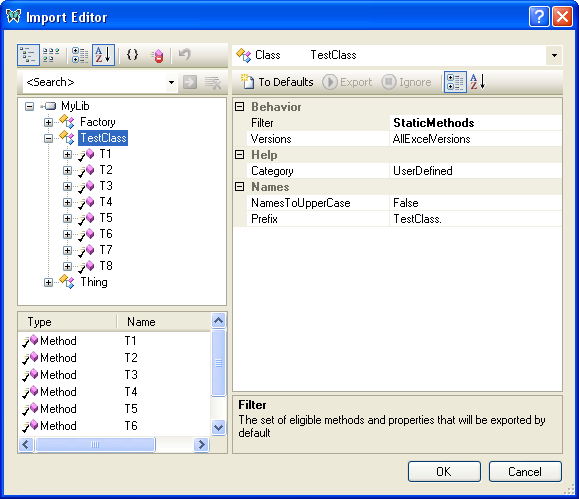3. Select the type "Factory". In the property grid, set the Filter value to "StaticMethods".

4. Expand the type "Factory", until you can see both of its public methods. Note that both methods have the same name, "CreateObject". C# supports overloading, but Excel does not. So, for at least one of these methods, we need to change the name exported to Excel.

Select the version of "CreateObject" which has no arguments, and, using the property grid, change its ExportedName to `Factory.CreateDefaultObject`.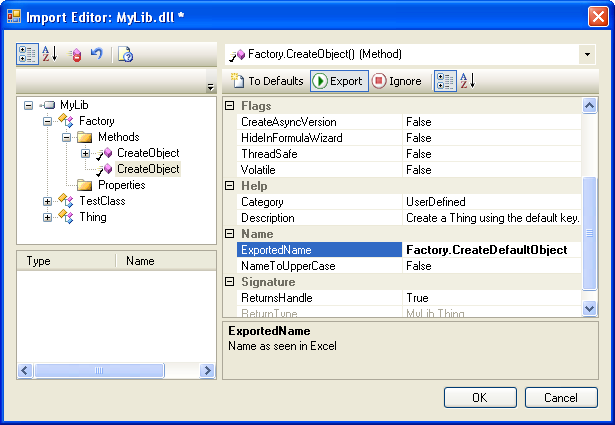5. Select the type "Thing", and set its Filter to "StaticMethods, InstanceMethods, InstanceProperties", by checking three boxes in the drop-down box, as shown below.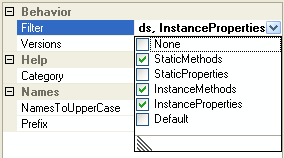6. Expand the type "Thing" until you can see all its methods. Select and expand the "DiscountFactor" method. Select the "period" argument.

In the property grid, set the ShowValueListInFormulaWizard property to `True`.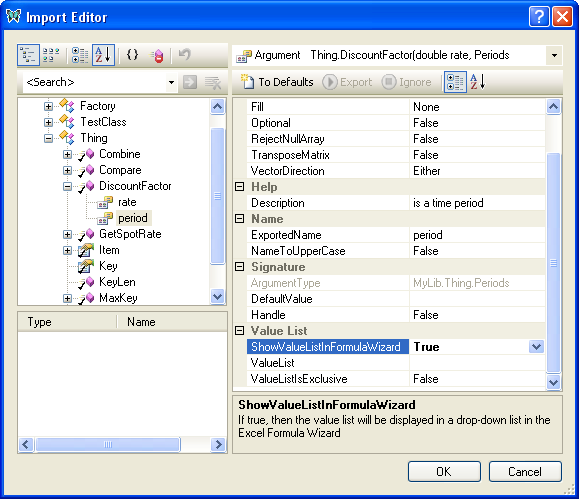Because "period" is an Enum, and the ShowValueListInFormulaWizard property has been set, a Value List will be created which will be displayed in the Excel Formula Wizard at run-time:7. In the tree view, select and expand the "GetSpotRate" method of the "Thing" class. Select the "ccy" argument.

In the property grid, set the ShowValueListInFormulaWizard property to `True`.

Also in the property grid, select the ValueList property and enter the following value:

AUD,Australian Dollar;EUR,Euro;GBP,Pound Sterling;JPY,Japanese Yen;USD,US Dollar

Optionally, you can then press the edit button (...) to show the Value List Editor.Press OK to close the Value List Editor.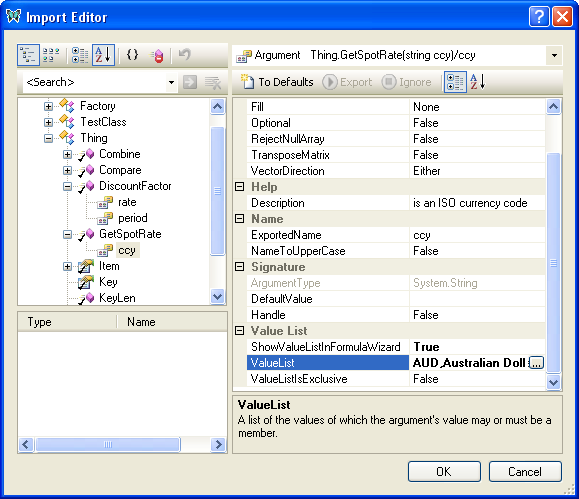At run-time, the value list will be displayed in the Excel Formula Wizard: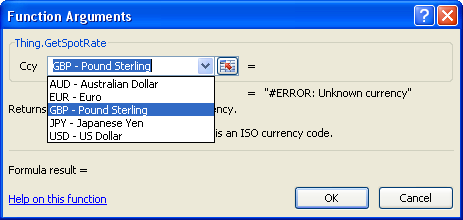8. Click OK to save your changes in the Import Editor.

9. Click OK in the Imported Assemblies window to save your changes and return to Visual Studio.

### The completed project

If you inspect the add-in project in Solution Explorer, you will see that two new files have been added. In the folder "NetWrap/Imported files" are `MyLib.import.xml` and `MyLib_Wrappers.cpp`.

`MyLib.import.xml` contains instructions for importing the .NET assembly. When it is built, the XLL+ reflection tool, XNREFLECT.EXE, is run, which generates the C++ code for the XLL add-in functions, and write it to `MyLib_Wrappers.cpp`.

1. Build the project. If you are working with a 64-bit version of Excel, then be sure to select the 64-bit platform `x64` before you build.

You will observe a new step in the build process, when `MyLib_Wrappers.cpp` is generated.

2. Inspect `MyLib_Wrappers.cpp`. Each of the functions is simply a wrapper for a .NET method, and contains code to validate inputs, translate them to CLR data forms, invoke the .NET method and finally convert the result to Excel data form. If any method throws an exception, it will be caught and reported back to Excel.

3. Use F5 to run and debug the add-in. Place break-points in the C# code, and you will see that the debugger seamlessly steps between the C++ and C# modules.

See Importing .NET assemblies in the User Guide for more details.